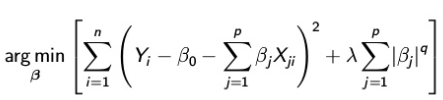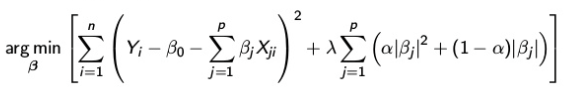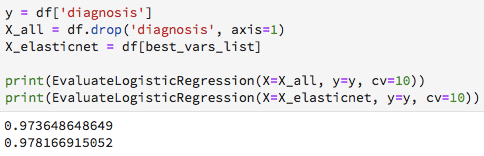# Regularised Logistic Regression

## Reduce the number of variables for breast cancer diagnosis

When dealing with many different variables to predict an outcome, it not only takes a lot more time to gather all the information, depending on the kind of experiment, you may also collect information that is not necessarily relevant or correlates with other variables. In this analysis I will use a technique that reduces the number of variables of a dataset, while it also improves the performance of the model, and therefore simplifies the model.

First, I will shortly explain the concept of this technique and then will provide an example to predict breast cancer diagnosis.

### Overcoming Overfitting with Regularization

When a true relationship between the response and the predictors is approximately linear, the OLS estimates from the model will have low bias: The difference between the expected (or average) prediction of your model and the correct value which you’re are trying to predict is low, and your model measures what it’s supposed to measure. If the number of observations is much larger than the number of variables, then the OLS estimates tend to have low variance, and hence will perform well on test observations: The predictions for a given observation will vary less between different realizations of the model, because there are few variables that influence the response.

As we add more explanatory variables to our model the OLS estimates for different samples tend to be much larger in magnitude than the true values, which is the same as high variance and even lower bias. The model becomes too flexible and there is no longer a unique OLS solution: The model overfits the data. As a consequence, you need to find the optimum point in your model where the decrease in bias is equal to increase in variance. Balancing this bias and variance is called the bias-variance trade-off.

There are two methods to overcome overfitting:

• Reduce the complexity of the model
• Regularization

Regularization puts a constraint on the size of coefficients. In a complex model there tends to be a larger difference between the size of coefficients. A large coefficient means that we’re putting a lot of emphasis on that feature. As a result, the algorithm starts modelling intricate relations to estimate the response. This problem can be amplified when features with high coefficients are also correlated. Therefore, putting a constraint on the magnitude of coefficients will also reduce model complexity. Moreover, by shrinking the estimated coefficients, we can often reduce the variance at the cost of a negligible increase in bias, improving the accuracy of the prediction for unseen observations.

There are two main types of regularization: Ridge and LASSO. Both add a penalty term to the loss function for having ‘large’ coefficients.

• For the Ridge regression: large with respect to the squared L2 norm (Euclidian distance) where q = 2
• For the LASSO regression: large with respect to the L1 norm (Manhattan distance) where q = 1Ridge (q=2) and LASSO (q=1) regression

Both have a tuning parameter lambda, which decides how important the penalty is in relation to the squared error term. The bigger the penalty, the higher the values of lambda, and therefore the magnitude of coefficients are reduced.

Ridge regression estimates are little affected by small changes in the data and when the predictor variables are highly multicollinear. The model will produce a different set of coefficient estimates for each value of lamba. As the value of lambda increases, the model complexity reduces. Though higher values of lambda reduce overfitting, significantly high values can cause underfitting as well. To balance the bias-variance trade-off it is therefore critical to select lambda wisely. A technique used to choose the optimal lambda is cross-validation. The idea behind cross-validation is that the value of lambda is iterated over a range of values and the value that minimizes the loss function is chosen as the best lambda. Though irrelevant coefficients for the best lambda are very small, they never become zero.

In contrast to Ridge regression, LASSO regression, which stands for Least Absolute Shrinkage and Selection Operator, functions as a feature selection by forcing the coefficients of irrelevant features to zero when the penalty is sufficiently large. An disadvantage of LASSO regression is that when we have correlated variables, it retains only one variable and sets other correlated variables to zero. This could lead to loss of information and result in lower accuracy of the model.

A hybrid model that is a combination of Ridge and LASSO can overcome this problem. An Elastic Net regression linearly combines L1 and L2 regularization of the Ridge and LASSO methods. As an addition to LASSO Elastic Net regression treats correlated features as a group. If any of the variables of a group of correlated features is a strong predictor, then the Elastic Net includes the entire group in the model.Elastic Net regression

### Elastic Net Regression Application

In this analysis I will explain how to use a regularised logistic regression with Scikit Learn to predict whether a breast cancer is benign or malignent.

The dataset contains of 30 features that are computed from a digitized image of a breast mass. Where 357 of the cancers where diagnosed as benign and 212 as malignant.

Lets find out whether we observe multicollinearity in the dataset to judge whether it would make sense to apply an Elastic Net regression.

We indeed see that around half of the variables are highly to medium correlated with another variable. In the case of this dataset it doesn’t take a lot of time to collect, or transform, the features. Instead, imagine that it would take significantly more time to collect information about each feature and feature selection is highly desired. In that case we will definitely want to explore the effect of an Elastic Net on the performance of the model.

First, I will explore the performance of the model for each combination of the lambda and Elastic Net. For this approach see the following steps. (Note with a regularised linear regression model you could use the ElasticNetCV function from Sklearn for this method. The SGDClassifier function for regularised logistics regression model does not return the same attributes and therefore requires a different method for cross-validation.)

Use the Bootstrap method to run the Elastic Net model ten times and each time store the coefficients from the model. At the end take the mean of the ten coefficients for each variable.

This creates a list of the most relevant features.

Compare the regular logistic regression model with the regularised Elastic Net logistic regression model.Evaluation of Logistic Regression with Elastic Net Logistic Regression

As these results show performing a regularised logistic regression model does not really improve the accuracy (ROC AUC score) of the model. A reason for this could be that the logistic regression already shows to be very accurate.

### Remarks

In this analysis we see that not performing variable selection through regularization is not be a problem of prediction accuracy. However, take a survey that contains hundreds of questions that are each asked to a respondent on the ground, a model that uses only a selection of the questions will not only make the model easier to interpret, it also saves a lot of time to run the experiment. In that case a regularised model would still be of huge benefit.